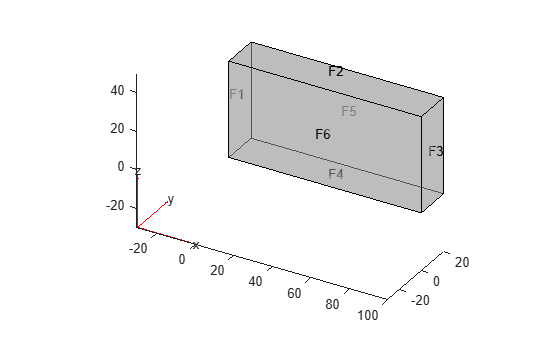# findBoundaryConditions

Find boundary condition assignment for a geometric region

## Syntax

``BCregion = findBoundaryConditions(BCs,RegionType,RegionID)``

## Description

example

````BCregion = findBoundaryConditions(BCs,RegionType,RegionID)` returns boundary condition `BCregion` assigned to the specified region.```

## Examples

collapse all

Create a PDE model and import a simple block geometry. Plot the geometry displaying the face labels.

```model = createpde(3); importGeometry(model,'Block.stl'); pdegplot(model,'FaceLabels','on','FaceAlpha',0.5)```Set zero Dirichlet conditions on faces 1 and 2 for all equations.

`applyBoundaryCondition(model,'dirichlet','Face',1:2,'u',[0,0,0]);`

On face 3, set the Neumann boundary condition for equation 1 and Dirichlet boundary condition for equations 2 and 3.

```h = [0 0 0;0 1 0;0 0 1]; r = [0;3;3]; q = [1 0 0;0 0 0;0 0 0]; g = [10;0;0]; applyBoundaryCondition(model,'mixed','Face',3,'h',h,'r',r,'g',g,'q',q);```

Set Neumann boundary conditions with opposite signs on faces 5 and 6 for all equations.

```applyBoundaryCondition(model,'neumann','Face',4:5,'g',[1;1;1]); applyBoundaryCondition(model,'neumann','Face',6,'g',[-1;-1;-1]);```

Check the boundary condition specification on face 1.

`findBoundaryConditions(model.BoundaryConditions,'Face',1)`
```ans = BoundaryCondition with properties: BCType: 'dirichlet' RegionType: 'Face' RegionID: [1 2] r: [] h: [] g: [] q: [] u: [0 0 0] EquationIndex: [] Vectorized: 'off' ```

Check the boundary condition specification on face 3.

`findBoundaryConditions(model.BoundaryConditions,'Face',3)`
```ans = BoundaryCondition with properties: BCType: 'mixed' RegionType: 'Face' RegionID: 3 r: [3x1 double] h: [3x3 double] g: [3x1 double] q: [3x3 double] u: [] EquationIndex: [] Vectorized: 'off' ```

Check the boundary condition specification on face 5.

`findBoundaryConditions(model.BoundaryConditions,'Face',5)`
```ans = BoundaryCondition with properties: BCType: 'neumann' RegionType: 'Face' RegionID: [4 5] r: [] h: [] g: [3x1 double] q: [] u: [] EquationIndex: [] Vectorized: 'off' ```

## Input Arguments

collapse all

Boundary conditions of a PDE model, specified as the `BoundaryConditions` property of `PDEModel`.

Example: `model.BoundaryConditions`

Geometric region type, specified as `'Face'` for 3-D geometry or `'Edge'` for 2-D geometry.

Example: `findBoundaryConditions(model.BoundaryConditions,'Face',3)`

Data Types: `char` | `string`

Geometric region ID, specified as a vector of positive integers. Find the region IDs by using `pdegplot` with the `'FaceLabels'` (3-D) or `'EdgeLabels'` (2-D) value set to `'on'`.

Example: `findBoundaryConditions(model.BoundaryConditions,'Face',3)`

Data Types: `double`

## Output Arguments

collapse all

Boundary condition for a particular region, returned as a `BoundaryCondition` object.

### Topics

Introduced in R2016b

## SupportGet trial now